# Depreciated Cost

Is the fixed asset's value minus all the depreciation that has been recorded against it

Depreciation is the accounting process of transforming the initial costs of fixed assets like plant and machinery, equipment, and other assets into expenses. It is the loss of value of fixed assets as a result of their use, the passage of time, or obsolescence.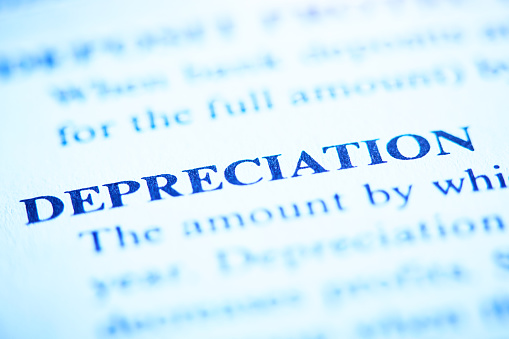Depreciation is also a non-cash expenditure because it does not entail any actual cash outflow. As a result, depreciation as a cost is distinct from all other types of expenses. Depreciation is the process by which the value of a company's assets depreciates over time.

In the United States, accountants must calculate and report depreciation on financial statements using generally accepted accounting standards (GAAP).

GAAP (Generally Accepted Accounting Principles) is a system of standards that governs the specifics, complexity, and legality of commercial and corporate accounting.

Depreciation is defined as the systematic reduction in the reported value of a fixed asset until the asset's value is zero or inconsequential in accounting terms.

Buildings, furniture, office equipment, machinery, and other fixed assets are examples of the same. The sole exception is land, which cannot be depreciated since its value increases with time.

Revenues are recorded with their corresponding costs in the accounting period when the asset is in use, this is required under the matching principle. This makes it easier to acquire a clearer view of the revenue generation transaction.

The fixed asset's value minus all the depreciation that has been recorded against it is called depreciated cost. In a larger economic sense, is the total amount of capital that is "used up" in a certain period.

It may be used to look for patterns in a company's capital investment and how aggressive its accounting techniques are, as measured by how precisely depreciation is calculated.

## Is depreciation a fixed cost or variable cost?

Depreciation is a fixed expense since it occurs at the same rate every period during the asset's useful life. Depreciation is not a variable expense because it does not change with the level of activity. There is, however, one exception.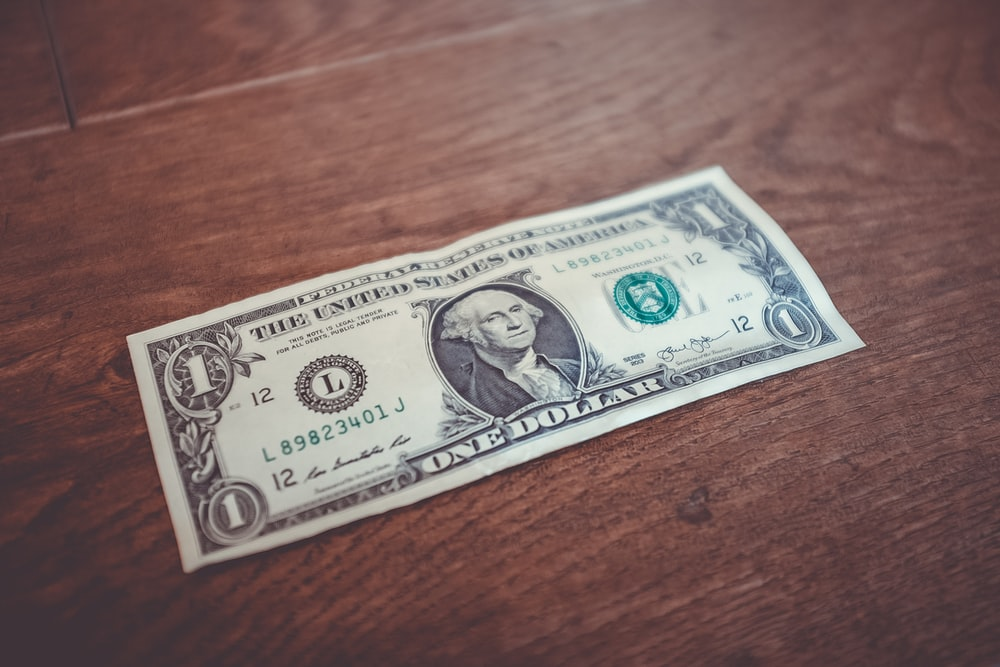Depreciation will be incurred in a manner that is more consistent with a variable cost if a company uses a usage-based depreciation technique.

A logging equipment, for example, is depreciated based on the number of hours it is utilized, therefore depreciation costs will vary depending on the number of trees chopped.

These trees are later sold to produce money, the resulting depreciation might be considered a variable rather than a fixed cost.

However, because usage-based depreciation schemes are uncommon, depreciation cannot be considered a variable cost in most circumstances.

If depreciation is considered a fixed cost, it is factored into the numerator of the method for calculating a company's break-even sales, which is:

Total fixed expenses ÷ Contribution margin % = Break even sales

Depreciation is used to lower the amount of the contribution margin percentage in the denominator of the calculation if it is deemed a variable expense, which may be claimed if usage-based depreciation is applied.

The operating and overhead costs of a firm are known as fixed costs. Items such as building, rent, utilities, labor, and insurance are included.

## Methods to calculate depreciation

When calculating depreciation, a corporation considers a variety of elements. The technique of depreciation is one such element. As a result, multiple depreciation methodologies are used by organizations to compute depreciation.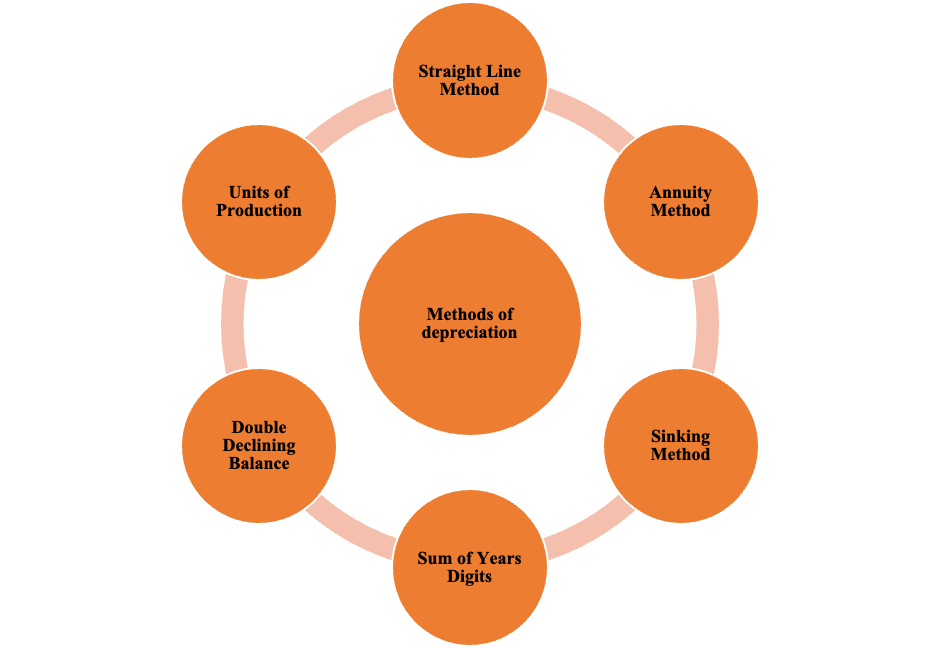Depreciation Methods

• Straight Line Depreciation Method
• Diminishing Balance Method
• Sum of Years' Digits Method
• Double Declining Balance Method
• Sinking Fund Method
• Annuity Method
• Insurance Policy Method
• Discounted Cash Flow Method
• Use Based Method
• Output Method
• Working Hours Method
• Mileage Method
• Other Methods
• Depletion Method
• Revaluation Method
• Group or Composite Method

As seen above, there are numerous methods to calculate depreciation, each way different from another in terms of how it's calculated and the items considered in the calculation.

For simplicity of understanding, we are only going to discuss the four most commonly used methods to calculate depreciation.

### 1)    Straight Line Depreciation Method

This is one of the most commonly used methods to compute depreciation. It is  also referred to as the fixed-installment approach. In this method, the depreciation of each fixed asset is charged at the same rate in each accounting period.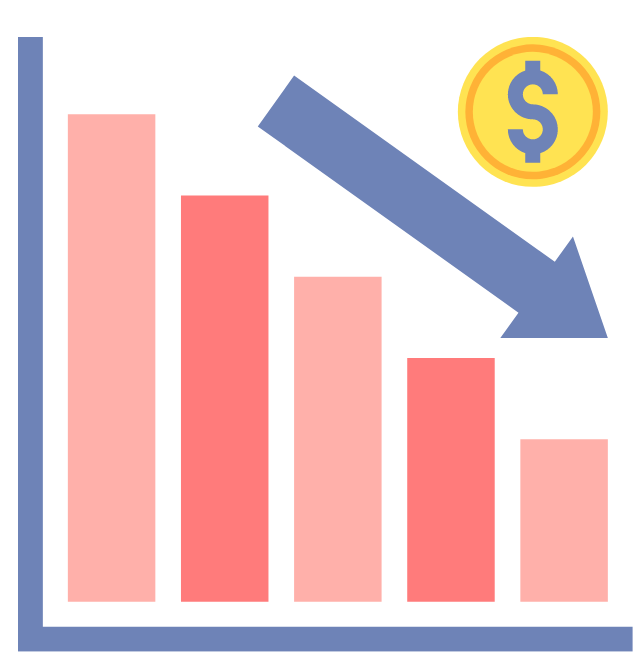This constant cost is charged until the asset is depreciated to zero or its salvage value at the end of its expected useful life.

As a result, the name of this approach comes from a straight-line graph. After charting an equal amount of depreciation for each accounting period during the asset's useful life, this graph is produced.

The depreciation is determined by dividing the difference between the original cost and the book value of the fixed asset.

The following is the calculation for yearly depreciation using the straight line method:

Annual Depreciation Expense = (Asset Cost – Salvage Value)/Asset Useful Life

• The asset's cost is either its acquisition price or its historical cost.
• The worth of an asset after it has outlived its useful life is referred to as salvage value.
• The amount of years for which an asset is projected to be employed by the firm is referred to as the asset's useful life.

### 2)    Diminishing Balance Method

This reducing balance approach is also known as the writing down value method or the declining balance method. Under this technique, a fixed percentage of depreciation is charged to the net balance of the fixed asset in each accounting period.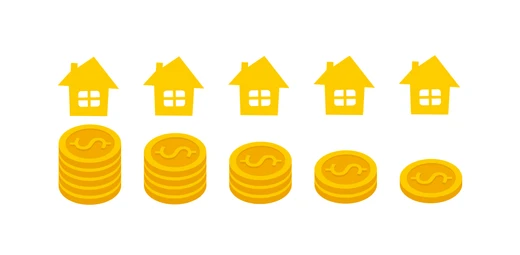After subtracting cumulative depreciation, the net balance represents the worth of the asset that remains.

As a result, the depreciation rate is applied to the asset's decreasing balance.

This is the asset that is recorded in the books of accounts at the start of the accounting period. As a result, the asset's book value is written down to decrease it to its residual value.

Every year, the amount of depreciation decreases as the asset's book value decreases. As a result, depreciation is charged at a greater rate in the early years of an asset compared to later stages.

As a result, the technique is predicated on the idea that higher depreciation should be charged in the asset's early years. This is since such years have minimal repair costs. The cost of repairs and upkeep of an asset rises as it enters the later phases of its useful life.

Depreciation Expense = (Book Value of Asset at Year's Start x Depreciation Rate)/100Begin your journey into Excel modeling with our free Excel Modeling Crash Course.

### 3)    Sum of Years' Digits Method

The Sum of Years' Digits Technique is another accelerated depreciation method. This strategy accelerates the recognition of depreciation.As a result, under this technique, the depreciable amount of an asset is charged as a  fraction across many accounting periods.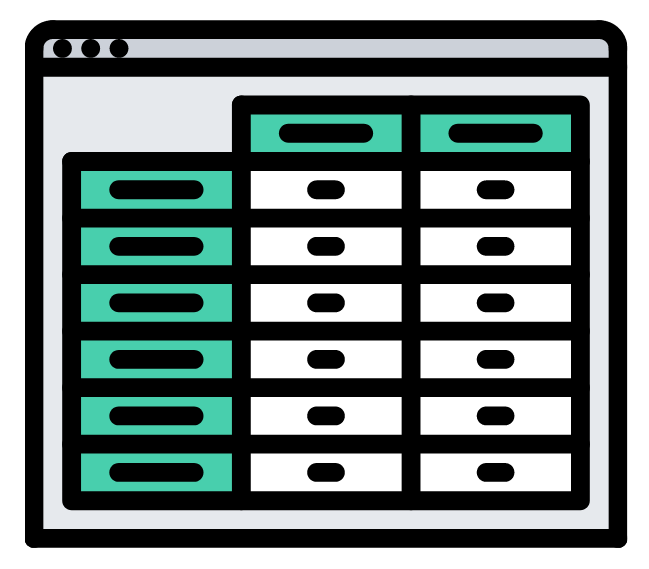This fraction is the ratio of an asset's remaining usable life in a certain time to the sum of the years' digits. As a result, this proportion implies that in the first year, the capital blocked or profit generated from the asset is the largest.

Therefore , as an asset approaches the end of its useful life, the benefit derived from it decreases. This is because no capital has been recovered until then, the largest amount of depreciation is given in the first year.

As a result, the last year should have the least amount of depreciation because most of the capital invested has been recovered.

### 4)    Double Declining Balance Method

This approach combines the straight line and declining balance techniques. As a result, under this technique, depreciation is charged on the lower value of the fixed asset at the start of the year.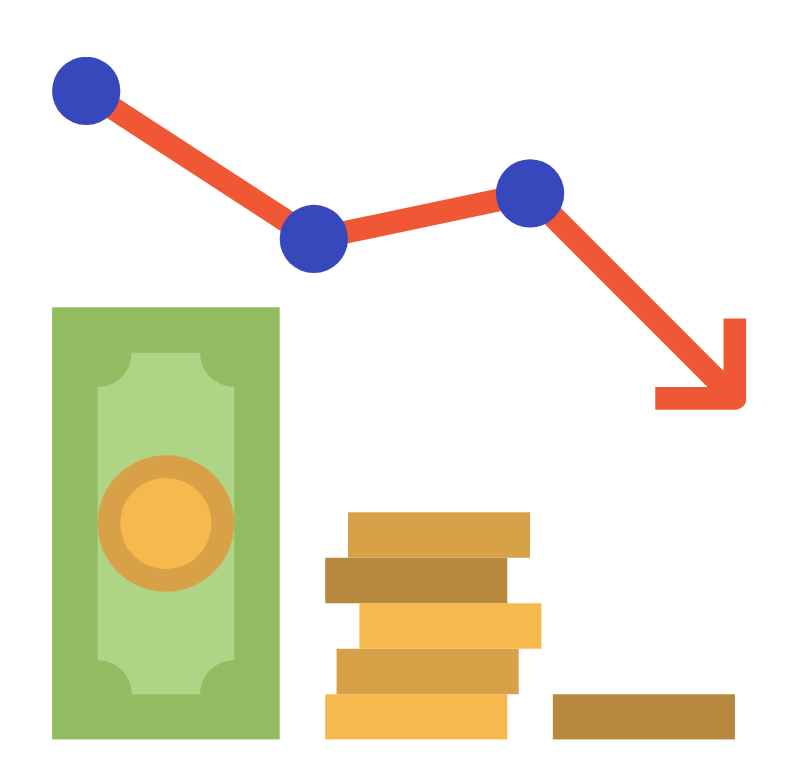This is similar to the approach of declining balance. However, much like the straight line technique, a set rate of depreciation is used.

This depreciation rate is twice as high as the rate paid under the straight line approach. As a result, when compared to the expected salvage value, this strategy results in an excessively depreciated asset at the end of its useful life.

As a result, firms take a variety of techniques to solve such a problem. The amount of depreciation charged for the previous year is first adjusted. This is done to bring the salvage value up to par with the expected salvage value.

Second, many corporations choose to modify the over depreciated salvage value using the straight line depreciation technique in the previous year.

The following is the formula:

Annual Depreciation Expense = 2 x (Cost of Asset – Salvage Value)/Useful Life of Asset Or Depreciation Expense = 2 x Cost of Asset x Depreciation Rate

## How Depreciated Cost Works?

The depreciated cost technique of asset valuation is an accounting approach for determining the usable value of an item used by corporations and individuals. It's vital to remember that it does not equal market value.

The market value of an item is its price, which is determined by supply and demand in the market.The depreciated cost is the value of an asset after its useful life has ended, which is depreciated over time.Because the value of an item is continuously  reduced  by computing the depreciation cost, the depreciated cost technique always permits accounting records to represent an asset at its current value.

The formula is as follows:

Depreciated Cost= Purchase Price (or Cost Basis)−CD

where:

• CD is equal to the Cumulative Depreciation

For example, if a construction business can sell an inoperable crane for \$5,000 in parts, the crane's depreciated cost or salvage value is \$5,000. If the corporation paid \$50,000 for the crane, the total amount depreciated during its useful life is \$45,000.

Assume the crane has a 15-year useful life. The corporation now has all of the information it requires to compute depreciation for each year. Straight-line depreciation is the simplest way.

This indicates that the quantity of appreciation has no slope, whether it's a 30 percent depreciation when driving new automobiles off the lot or a higher depreciation when an item is nearing the point of needing substantial repairs.

Depreciation is the same every year when using this strategy. It's calculated by dividing total depreciation (\$45,000) by the useful life (15 years), which comes to \$3,000 each year.Depreciated Cost = Original Cost - Accumulated Depreciation

### Factors for Estimating Depreciation

In order to determine the amount of depreciation to be charged in each accounting period, a number of elements must be taken into account.These are some of them:

1.  Cost of the Asset

The historical cost is another name for the asset's cost. It includes the fixed asset's acquisition price as well as any additional expenditures necessary to get the asset up and running. Freight and shipping, installation charges, commission, insurance, and so on are all included in these prices.

1.  Salvage Value

Salvage value is also known as scrap value or net residual value. It refers to an asset's anticipated net realizable value at the end of its useful life. The difference between the sale price and the costs required to dispose of an item determines this value.

1. Estimated Useful Life

The useful life of an asset is defined as its commercial or economic life. The physical life of an asset is no longer taken into account when determining its useful life. This is due to the fact that an asset may be in good physical shape after a few years, yet it may not be employed for production.

1. Method of Depreciation

You must develop an appropriate method for allocating the asset's cost over the asset's usage periods. In general, the method of depreciation to be utilized is determined by the predicted benefits derived from a certain item.

As a result, different strategies would be used for different sorts of assets in a business.

In practice, however, corporations do not consider service benefit patterns when deciding on a depreciation approach. In most cases, a single technique is used to depreciate all of the company's depreciable assets.

## Key Takeaways

• The value of a fixed asset less all the depreciation that has been recorded against it is called depreciated cost.
• It is used to determine the worth of an item after its useful life has ended.
• Companies may use the depreciated cost to evaluate their capital spending patterns as well as their accounting methods.
• The "salvage value," "net book value," or "adjusted cost base" are all terms for the depreciated cost.
• The depreciated cost method of asset valuation is an accounting method for evaluating the usable worth of a product used by firms and people.
• Depreciation is a fixed cost since it happens at the same amount across the asset's useful life. Because depreciation sometimes doesn't alter with the degree of operation, it is not a variable expenditure.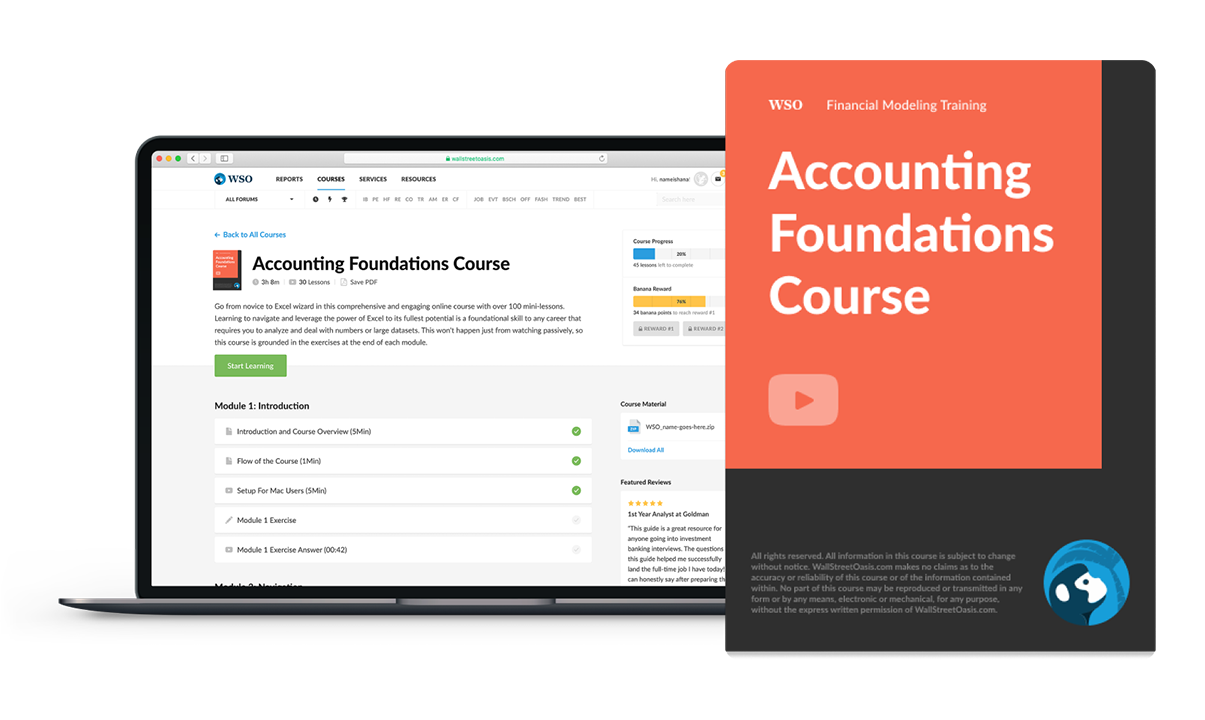### Everything You Need To Master Accounting Foundations

To Help you Thrive in the Most Prestigious Jobs on Wall Street.